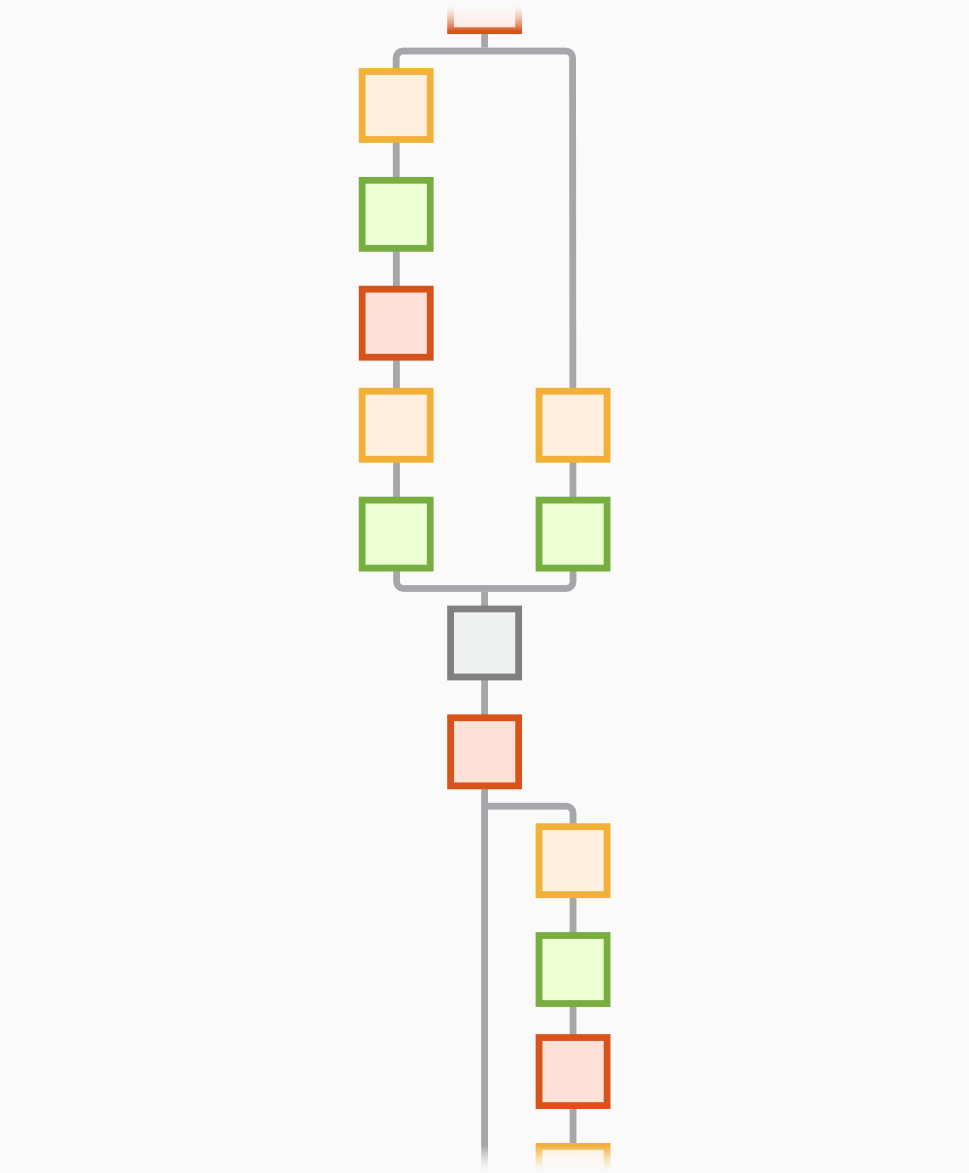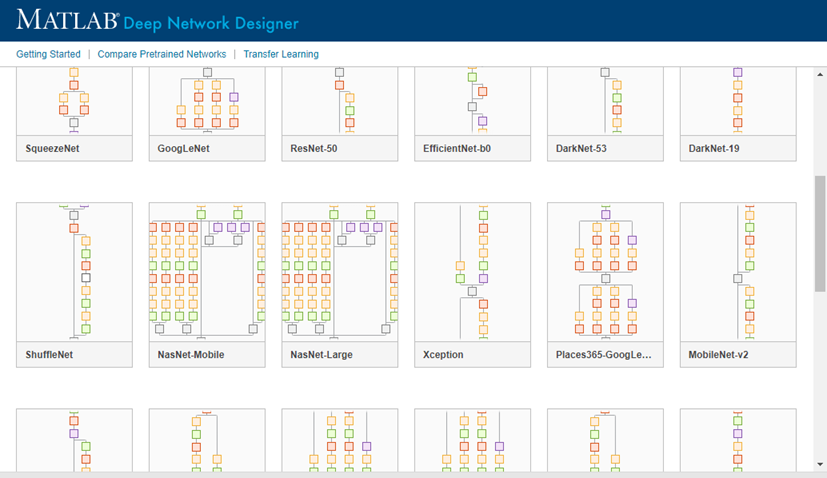# efficientnetb0

EfficientNet-b0 convolutional neural network

•## Syntax

``net = efficientnetb0``
``net = efficientnetb0('Weights','imagenet')``
``lgraph = efficientnetb0('Weights','none')``

## Description

EfficientNet-b0 is a convolutional neural network that is trained on more than a million images from the ImageNet database . The network can classify images into 1000 object categories, such as keyboard, mouse, pencil, and many animals. As a result, the network has learned rich feature representations for a wide range of images. The network has an image input size of 224-by-224. For more pretrained networks in MATLAB®, see Pretrained Deep Neural Networks.

You can use `classify` to classify new images using the EfficientNet-b0 model. Follow the steps of Classify Image Using GoogLeNet and replace GoogLeNet with EfficientNet-b0.

example

````net = efficientnetb0` returns an EfficientNet-b0 model network trained on the ImageNet data set.This function requires the Deep Learning Toolbox™ Model for EfficientNet-b0 Network support package. If this support package is not installed, then the function provides a download link.```
````net = efficientnetb0('Weights','imagenet')` returns a EfficientNet-b0 model network trained on the ImageNet data set. This syntax is equivalent to `net = efficientnetb0`.```
````lgraph = efficientnetb0('Weights','none')` returns the untrained EfficientNet-b0 model network architecture. The untrained model does not require the support package. ```

## Examples

collapse all

Download and install the Deep Learning Toolbox Model for EfficientNet-b0 Network support package.

Type `efficientnetb0` at the command line.

`efficientnetb0`

If the Deep Learning Toolbox Model for EfficientNet-b0 Network support package is not installed, then the function provides a link to the required support package in the Add-On Explorer. To install the support package, click the link, and then click . Check that the installation is successful by typing `efficientnetb0` at the command line. If the required support package is installed, then the function returns a `DAGNetwork` object.

`efficientnetb0`
```ans = DAGNetwork with properties: Layers: [290×1 nnet.cnn.layer.Layer] Connections: [363×2 table] InputNames: {'ImageInput'} OutputNames: {'classification'}```

Visualize the network using Deep Network Designer.

`deepNetworkDesigner(efficientnetb0)`

Explore other pretrained networks in Deep Network Designer by clicking .If you need to download a network, pause on the desired network and click to open the Add-On Explorer.

## Output Arguments

collapse all

Pretrained EfficientNet-b0 convolutional neural network, returned as a `DAGNetwork` object.

Untrained EfficientNet-b0 convolutional neural network architecture, returned as a `LayerGraph` object.

 ImageNet. http://www.image-net.org

 Mingxing Tan and Quoc V. Le, “EfficientNet: Rethinking Model Scaling for Convolutional Neural Networks,” ArXiv Preprint ArXiv:1905.1194, 2019.